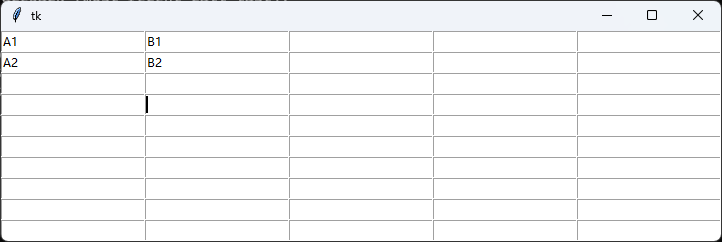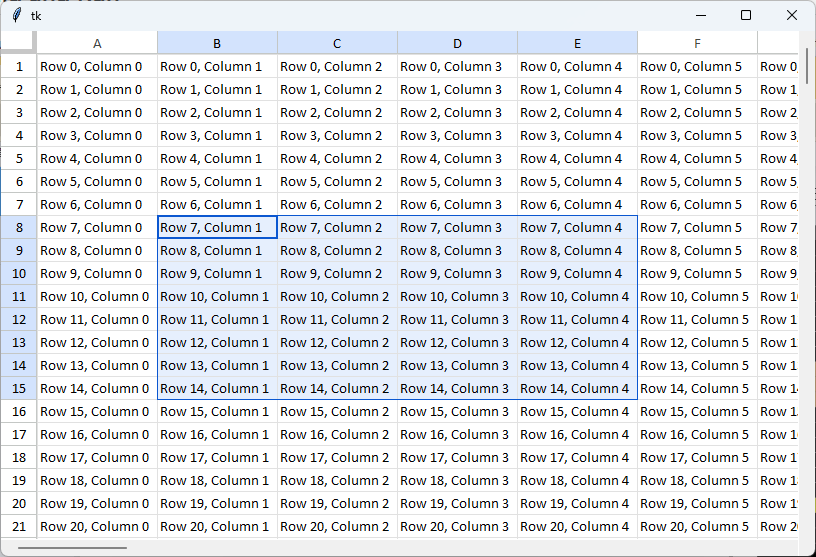# Python Tkinter Table Component

## Requirement

I want to add a table to a Python GUI program using tkinter. Since the official library does not provide a table component, I either need to find a community-made component or create my own table component.

## Approach One

Create a simple table using the grid layout feature. The downside is that it might not be very convenient to integrate with other components for GUI construction.### Usage

``````from tkinter import *

root = Tk()

height = 10
width = 5
cells = {}
for i in range(height): # Rows
for j in range(width): # Columns
b = Entry(root, text="")
b.grid(row=i, column=j)
cells[(i, j)] = b

mainloop()
``````

## Approach Two

Utilize the community-built component "tksheet," which is a table manually drawn using tkinter canvas.GitHub Repository: https://github.com/ragardner/tksheet

It supports rendering large datasets with potentially hundreds of millions of cells by only redrawing the visible portion of the table, ensuring smooth performance. It also supports cell colors and backgrounds.

### Usage

Make sure you have Python 3.6+ installed and install the dependency:

``````pip install tksheet
``````

Usage example:

``````from tksheet import Sheet
import tkinter as tk

class Demo(tk.Tk):
def __init__(self):
tk.Tk.__init__(self)
self.grid_columnconfigure(0, weight=1)
self.grid_rowconfigure(0, weight=1)
self.frame = tk.Frame(self)
self.frame.grid_columnconfigure(0, weight=1)
self.frame.grid_rowconfigure(0, weight=1)
self.sheet = Sheet(self.frame,
data=[[f"Row {r}, Column {c}\nnewline1\nnewline2" for c in range(50)] for r in range(500)])
self.sheet.enable_bindings()
self.frame.grid(row=0, column=0, sticky="nswe")
self.sheet.grid(row=0, column=0, sticky="nswe")

app = Demo()
app.mainloop()
``````